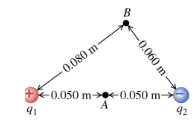# Problem: Two point charges q1 = 2.00 nC and q2 = -6.20 nC are 0.100 m apart. Point A is midway between them; point B is 0.080 m from q1 and 0.060 m from q2 (the figure ). Take the electric potential to be zero at infinity.a. Find the potential at point A. b. Find the potential at point B. c. Find the work done by the electric field on a charge of 2.00 nC that travels from point B to point A.

###### FREE Expert Solution

The electric potential is expressed as:

$\overline{){\mathbf{V}}{\mathbf{=}}\frac{\mathbf{k}\mathbf{q}}{\mathbf{r}}}$

Wowk done by electric field:

$\overline{){\mathbf{W}}{\mathbf{=}}{\mathbf{q}}{\mathbf{∆}}{\mathbf{V}}}$

a.

VA = V1A + V2A

80% (346 ratings)###### Problem Details

Two point charges q1 = 2.00 nC and q2 = -6.20 nC are 0.100 m apart. Point A is midway between them; point B is 0.080 m from q1 and 0.060 m from q2 (the figure ). Take the electric potential to be zero at infinity.a. Find the potential at point A.

b. Find the potential at point B.

c. Find the work done by the electric field on a charge of 2.00 nC that travels from point B to point A.